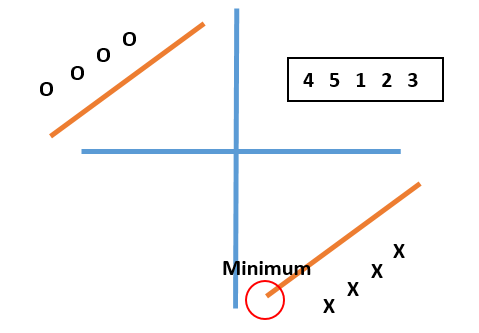So given a rotated sorted array, find the minimum value of the element in the array.

For `[4, 5, 1, 2, 3]`, return `1`.
For `[4,5,6,7,0,1,2]`, return `0`.

So the idea is to perform a binary search on the array and set the target as the last element. Then we perform a binary search and ensure that the target is going towards the minimum value, then we return its corresponding index.``````public int findMin(int[] nums){
if(nums == null || nums.length == 0){
return -1;
}

int start = 0, end = nums.length - 1;

while (start + 1 < end){
int mid = start + (end - start) / 2;

if(nums[mid] == nums[end]){
end = mid;
} else if(nums[mid] > nums[end]){
start = mid;
} else {
end = mid;
}
}

if(nums[start] <= nums[end]){
return nums[start];
}

return nums[end];
}
``````

When the mid value is smaller or equal to the min value, we need to move the end pointer to be mid pointer because we might find a smaller value throughout the process. Likewise, when the mid value is greater than min value, we need to move the start pointer to mid pointer.

#### What if the array contains duplicate? Can the algorithm still works?

The logic here needs to slightly change. Instead of using the last element as the min value, we move the end pointer forward when the mid value is equal to end value. The smallest element will not be removed.

``````        ...

if(nums[mid] == nums[end]){
end--;
} else if(nums[mid] > nums[end]){
start = mid;
} else {
end = mid;
}

...
}
``````

#### What about finding max value in sorted array?

Well, in that case, I will use first element as target and revert the logic of getting minimum in previous example:

``````...

while(start + 1 < end){
int mid = start + (end - start) / 2;
if(nums[mid] == nums[start]){
start = mid;  // going backward
} else if(nums[mid] < nums[start]){
end = mid;
} else {
start = mid;
}
}

if(nums[end] >= nums[start]){
return nums[end];
} else{
return nums[start];
}
...

``````

There you have it! I hope these two examples will help you understand better how to find minimum or maximum value in a rotated search array using binary search.

Post was published on , last updated on .

Like the content? Support the author by paypal.me!